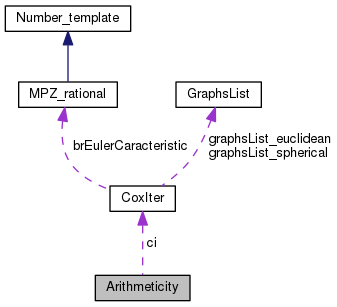CoxIter  1.2 CoxIter - Computing invariants of hyperbolic Coxeter groups
Arithmeticity Class Reference

This class tests the arithmeticity of a graph which has no dotted edge and which is non-cocompact. It uses Vinberg's criteria. More...

`#include <arithmeticity.h>`

Collaboration diagram for Arithmeticity:[legend]

## Public Member Functions

Arithmeticity ()
Basic constructor. More...

~Arithmeticity ()
Destructor. More...

void test (CoxIter &ci, const bool &bListCycles_)
Test the arithmeticity of a graph. More...

vector< string > get_strListCycles ()
Return the list of cycles. More...

string get_strError ()
Return the error code. More...

## Private Member Functions

unsigned int collapseQueues ()
Try to collapse queues of the graph. More...

void testCycles ()
Test the cycles. More...

void findCycles (const unsigned int &iRoot, const unsigned int &iFrom)
Look for cycles. More...

void testCycle ()
Test the cycle in iPath. More...

## Private Attributes

string strError
If an error occured, small text. More...

CoxIterci
Pointer to the CoxIter object. More...

unsigned int iVerticesCount
Number of generators of the group. More...

vector< vector< unsigned int > > iCoxeterMatrix
Coxeter matrix of the group. More...

vector< unsigned int > iReferencesToLabels
Correspondence for the new indices to the old ones. More...

vector< vector< bool > > bEdgesVisited
Traversed edges. More...

vector< bool > bVerticesVisited
Taversed vertices. More...

vector< unsigned int > iPath
Current path. More...

bool bNotArithmetic
True if not arithmetic (i.e. we have to quit the algorithm) More...

bool bListCycles
If true, will list the cycles to be manually tested. More...

vector< string > strListCycles
The list. More...

## Detailed Description

This class tests the arithmeticity of a graph which has no dotted edge and which is non-cocompact. It uses Vinberg's criteria.

## Constructor & Destructor Documentation

 Arithmeticity::Arithmeticity ( )

Basic constructor.

 Arithmeticity::~Arithmeticity ( )

Destructor.

## Member Function Documentation

 Arithmeticity::collapseQueues ( )
private

Try to collapse queues of the graph.

Returns
Number of vertices removed
 Arithmeticity::findCycles ( const unsigned int & iRoot, const unsigned int & iFrom )
private

Look for cycles.

Update the vector iPath to find cycles

Parameters
 iRoot( unsigned int& ) Starting vertex iFrom( unsigned int& ) Previoud vertex (if recursive call); iRoot otherwise
 Arithmeticity::get_strError ( )

Return the error code.

Returns
Error code (string)
 Arithmeticity::get_strListCycles ( )

Return the list of cycles.

Returns
List (vector<string>)
 Arithmeticity::test ( CoxIter & ci, const bool & bListCycles_ )

Test the arithmeticity of a graph.

Parameters
 ci( CoxIter& ) The graph bListCycles_( const bool& ) If true, will list the cycles to be manually tested
Returns
True if success, false otherwise. Then, use ci.get_iIsArithmetic( )
 Arithmeticity::testCycle ( )
private

Test the cycle in iPath.

This function is called by findCycles. Eventually, set bNotArithmetic to true

 Arithmeticity::testCycles ( )
private

Test the cycles.

## Member Data Documentation

 vector< vector< bool > > Arithmeticity::bEdgesVisited
private

Traversed edges.

 bool Arithmeticity::bListCycles
private

If true, will list the cycles to be manually tested.

 bool Arithmeticity::bNotArithmetic
private

True if not arithmetic (i.e. we have to quit the algorithm)

 vector< bool > Arithmeticity::bVerticesVisited
private

Taversed vertices.

 CoxIter* Arithmeticity::ci
private

Pointer to the CoxIter object.

 vector< vector< unsigned int > > Arithmeticity::iCoxeterMatrix
private

Coxeter matrix of the group.

 vector< unsigned int > Arithmeticity::iPath
private

Current path.

 vector< unsigned int > Arithmeticity::iReferencesToLabels
private

Correspondence for the new indices to the old ones.

 unsigned int Arithmeticity::iVerticesCount
private

Number of generators of the group.

 string Arithmeticity::strError
private

If an error occured, small text.

 vector< string > Arithmeticity::strListCycles
private

The list.

The documentation for this class was generated from the following files: